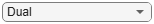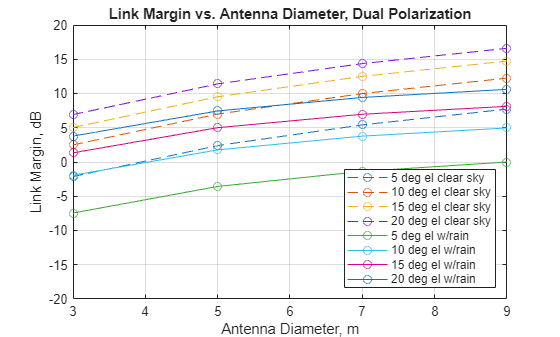# Antenna Size Analysis Using ITU-R P.618 Propagation Model

### Introduction

This example shows how to select a parabolic antenna diameter for a particular ground location using the ITU P.618  propagation model function in the Satellite Communications Toolbox. The appropriate antenna size may be quite sensitive to local climate conditions, such that locations with rainy climates will require larger antennas than those with dry climates. The ITU-R P.618 recommendation provides a comprehensive model for attenuation, noise temperature, and depolarization of radio signals through the atmosphere for space-to-ground links in every area of the earth.

This example uses the Satellite Communications Toolbox P.618 propagation model function. The example particularly applies to low earth orbit (LEO) satellites as they traverse a range of elevation angles on each pass.

### Obtain Map Data Required by P.618

Download and unpack ITU data maps if the zipped map file is not already present on the MATLAB® path. If the zipped map file already exists on the MATLAB path, just unpack it.

```maps = exist('maps.mat','file'); p836 = exist('p836.mat','file'); p837 = exist('p837.mat','file'); p840 = exist('p840.mat','file'); matFiles = [maps p836 p837 p840]; if ~all(matFiles) if ~exist('ITURDigitalMaps.tar.gz','file') url = 'https://www.mathworks.com/supportfiles/spc/P618/ITURDigitalMaps.tar.gz'; websave('ITURDigitalMaps.tar.gz',url); untar('ITURDigitalMaps.tar.gz'); else untar('ITURDigitalMaps.tar.gz'); end addpath(cd); end```

### Specify System Parameters

Specify the range of ground antenna diameters and elevation angles to be analyzed. An outage percentage of 1% is chosen, which corresponds to an availability of 99%.

Singapore is chosen for the ground station site as it has a high rain rate when compared to the rest of the world.

Other parameters specified are satellite equivalent isotropic radiated power (EIRP), satellite altitude, satellite transmit frequency, ground station antenna efficiency, ground station radome loss, pointing loss, receiver temperature, implementation loss, required Es/No, and either single or dual polarization.

```mgnParams.pctOutage = 1; % Outage percentage; % Availability = (100 - p) percent mgnParams.gndAntLat = 1.29; % North latitude, deg mgnParams.gndAntLon = 103.5; % East longitude, deg mgnParams.satEIRP = 27.0; % Satellite EIRP, dBW mgnParams.satAlt = 500; % Satellite altitude, km mgnParams.satTransmitFreq = 8.2; % Satellite transmit frequency, GHz mgnParams.antEff = 0.631; % Ground station antenna efficiency mgnParams.radomeLoss = 0.74; % Radome loss, dB mgnParams.ptgLoss = 0.2; % Pointing loss, dB mgnParams.rcvrTemp = 100; % Receiver noise temperature, K mgnParams.symRate = 100e6; % Symbol rate, sym/sec mgnParams.implLoss = 2.5; % Implementation loss, dB mgnParams.EsNoRqd = 17.8; % Required Es/No for BER=1e-5 in % AWGN-only channel, dB % 12.6 for OQPSK, 20.6 for D8PSK, % 17.8 dB for 8PSK gndAntDiam = [3 5 7 9]; % Ground antenna diameters, m gndAntEl = [5 10 15 20]; % Elevation angles, deg satAntPol ="Dual"; % Antenna polarization % Initialize outputs [marginSinglePol,marginDualPol] = deal(zeros(size(gndAntDiam))); linkMarginVsElAng = zeros(length(gndAntDiam),length(gndAntEl)); lgndEntries = repmat("",numel(gndAntEl)*2,1); % *2 for clear sky and rain```

### Run the Analysis

This code section calls HelperLinkMarginVsEl, which calls the MATLAB function `p618PropagationLosses` for each set of elevation angles and antenna diameters. These results are then plotted to clearly illustrate the link margins available for various configurations and geometries.

Note that both clear sky and with rain results are produced. This plot helps to clearly distinguish the performance under the best and worst case rain conditions for the given location and availability percentage.

```for clrSky = ["y" "n"] if clrSky == "y" clrSkyMkr = "--o"; lgndSkyStr = "clear sky"; lgndIdx = 0; else % "n" clrSkyMkr = "-o"; lgndSkyStr = "w/rain"; lgndIdx = 1; end numAntEl = length(gndAntEl); for idxEl = 1:numAntEl for idxDiam = 1:length(gndAntDiam) [marginSinglePol(idxDiam),marginDualPol(idxDiam)] = ... HelperLinkMarginVsEl(mgnParams,gndAntDiam(idxDiam), ... gndAntEl(idxEl),clrSky); end if satAntPol == "Dual" margin = marginDualPol; elseif satAntPol == "Single" margin = marginSinglePol; end plot(gndAntDiam,margin,clrSkyMkr); xlabel("Antenna Diameter, m"); ylabel("Link Margin, dB"); title("Link Margin vs. Antenna Diameter, " + satAntPol + ... " Polarization"); hold on; lgndEntries(numAntEl*lgndIdx+idxEl) = ... string(gndAntEl(idxEl)) + " deg el " + lgndSkyStr; % Accumulate columns into a matrix linkMarginVsElAng(:,idxEl) = margin; end end legend(lgndEntries(1:end), ... "Location","Southeast"); grid on; ylim([-20 20]);```For low elevation angles (5 and 10 degrees), it is difficult to close the link with an antenna size of less than 6 meters when rain falls at an annual 1 percent exceedance rate. For clear sky situations (no rain present), a 6 meter antenna would have almost zero margin at 5 degrees elevation. For additional margin, therefore, a diameter larger than 6 meters must be chosen.

### Generate Tabular Output

A tabular output of the matrix of link margins from the with rain analysis is computed and displayed. Each row corresponds to the analysis of one antenna diameter. Each column corresponds to the link margins at a given elevation angle for the various antenna diameters.

A possible application for such a table would be finding a polynomial fit for the margins at each elevation angle which are indicated by the columns in the table. Then the antenna diameter at each elevation angle for a particular margin (for example, 3 dB) value could be found. This assists in choosing a minimum elevation angle for a particular choice of antenna diameter.

```AntennaDiameter = gndAntDiam'; Margin5Deg = linkMarginVsElAng(:,1); Margin10Deg = linkMarginVsElAng(:,2); Margin15Deg = linkMarginVsElAng(:,3); Margin20Deg = linkMarginVsElAng(:,4); T = table(AntennaDiameter,Margin5Deg,Margin10Deg,Margin15Deg,Margin20Deg)```
```T=4×5 table AntennaDiameter Margin5Deg Margin10Deg Margin15Deg Margin20Deg _______________ __________ ___________ ___________ ___________ 3 -10.101 -3.6207 0.012847 2.6489 5 -5.9608 0.30741 3.8668 6.4839 7 -3.4614 2.5567 6.0346 8.6315 9 -1.7944 3.9742 7.3766 9.9556 ```

### Further Exploration

This example analyzes the location of Singapore and a satellite in a 500 km altitude circular orbit. Adjust the parameters in the function call to the helper function HelperLinkMarginVsEl and explore different ground station locations with different environmental parameters. These parameters are computed automatically by the MATLAB `p618PropagationLosses` function, or you can adjust any of the P.618 parameters manually. You can also adjust the altitude of the satellite, the carrier frequency, satellite EIRP, antenna diameters, system losses, receiver temperature, and required Es/No as desired.

The example showcases the dual polarization case. You can change the `satAntPol` variable to `single`, rerun the analysis, and compare the results to the dual polarized case.

Another important parameter is the annual exceedance percentage `(p)`, which is the probability that a particular rain rate and atmospheric noise temperature will be exceeded. Annual exceedance percentage relates to availability (`a`) as

`a` = (100 - `p`) %.

For LEO systems, the availability value has a less concrete meaning than for the geostationary orbit (GSO) case, where the geometry between the ground station and satellite is fixed. But as a qualitative measure, setting the availability to 99% is a typical value to use when assessing ground sites with moderate rain rates.

The example could be modified to address the GSO case by using the Satellite Communications Toolbox function `aer` in conjunction with a satellite scenario to compute the slant range between a ground station and a GSO satellite at a particular longitude. In this case the HelperLinkMarginVsEl function would have to be modified accordingly.

### Appendix

This example uses these helper functions:

### References

 International Telecommunication Union, ITU-R Recommendation P.618 (12/2017).